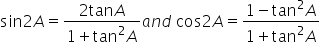Maths-
General
Easy

###Hint:

In the question we will first use the formula cos(A+B)=cosA.cosB-sinA.sinB and cos(A-B)=cosA.cosB+sinA.sinB then we will convert sin and cos in terms of tan using the formulathen we will make a quadratic equation assuming x in terms of tan. Then we will use the concept of D is greater than 0 to get the required result.

## Book A Free Demo+91